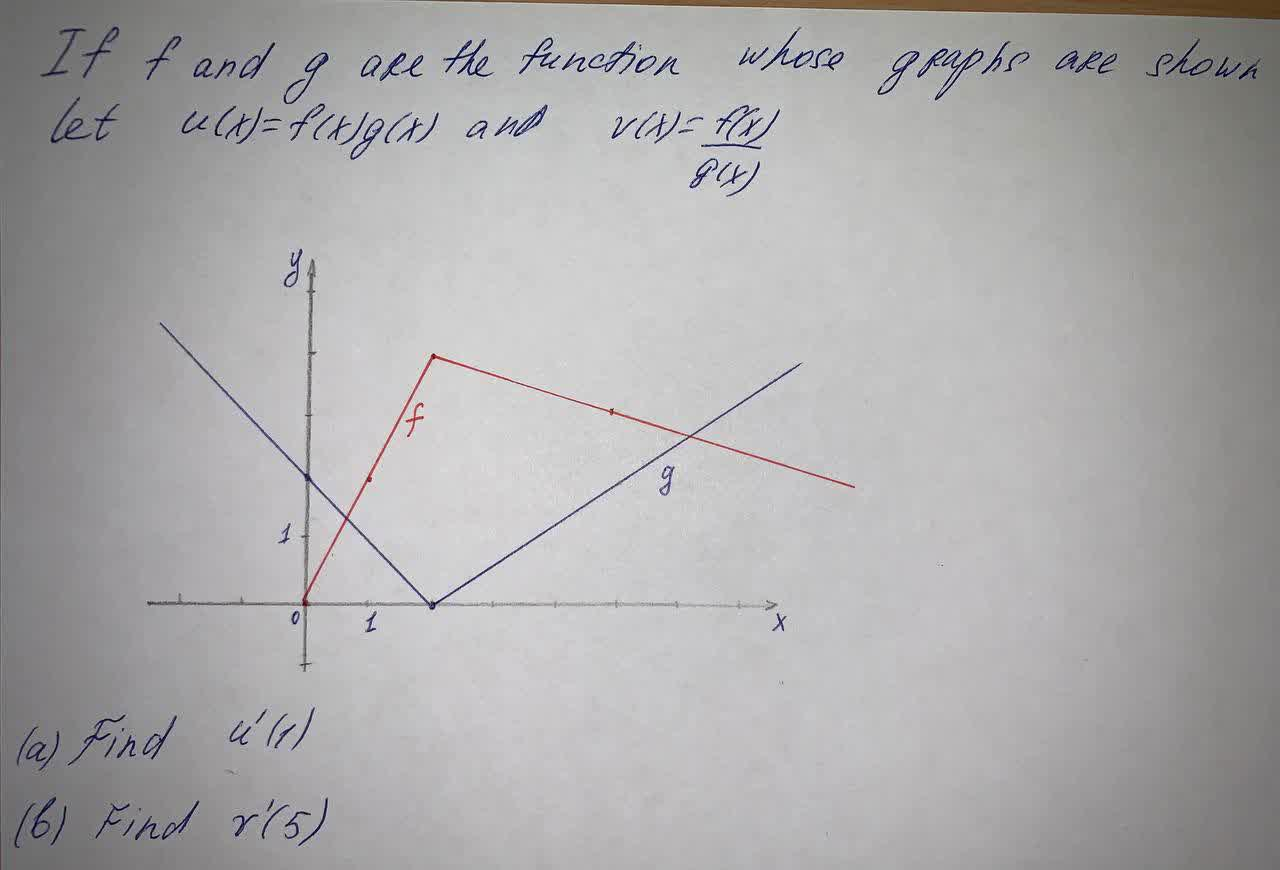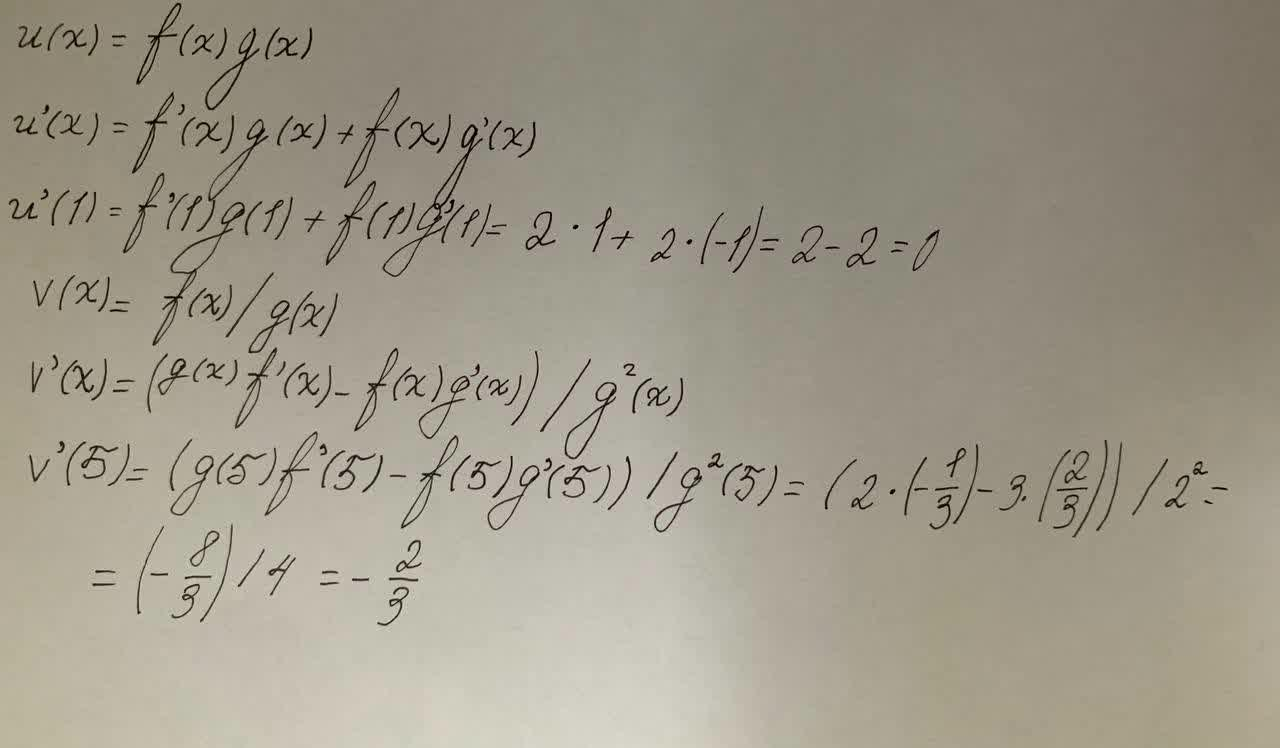#### Didn’t find what you are looking for?

Question# If f and g are the function whose graph are shown let u(x)= f(x)g(x) and v(x)=

Integrals
ANSWEREDIf f and g are the function whose graph are shown let $$u(x)= f(x)g(x)\ and\ v(x)=$$